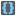• # Not a clue

## Question related to missionBrackets

Ok, so I started with this:

```LEFT = '{[('
RIGHT = '}])'

def checkio(expression):
l_brackets = []
r_brackets = []
for i in expression:
if i in LEFT:
l_brackets.append(i)
elif i in RIGHT:
r_brackets.append(i)
for l, r in zip(l_brackets, r_brackets[::-1]):
lc, rc = ord(l), ord(r)
if lc != rc-1 and lc != rc-2:
return False
return True
```

This worked for the first few examples, but then it fails with the expression checkio("[1+1]+(2*2)-{3/3}"). Now, I am clueless. That one expression renders my entire code useless. Any clues for me? Because this is doing my head in!9